# Problems of the Week

Contribute a problem

# 2017-05-22 Basic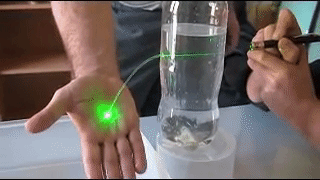A hole is pierced in the side of a water-filled bottle, so that water streams out as shown above.

When a laser beam is aimed toward the hole, the beam is seen to travel along the curved path of the water stream. What can explain this phenomenon?

True or False?

If I toss a fair coin once, then the only possible outcomes are heads or tails with equal probabilities. So the probability of obtaining a head from a single toss is $\frac12$.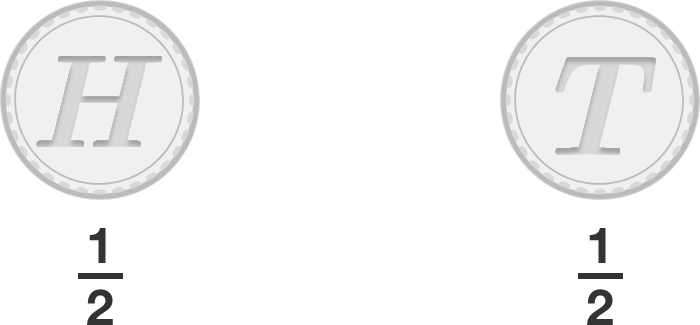If I toss a fair coin twice, then the only possible outcomes are "2 heads," "2 tails" or "1 head and 1 tail," again with equal probabilities. So the probability of obtaining "1 head and 1 tail" from two tosses is $\frac13$.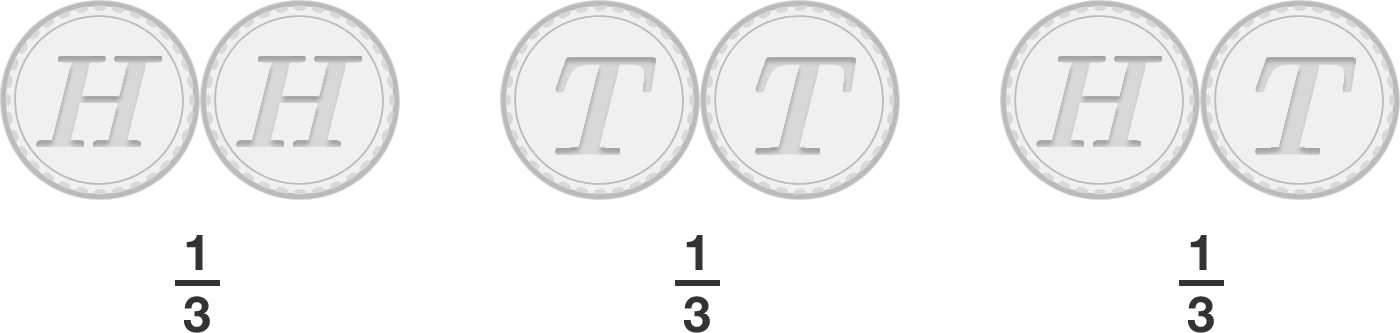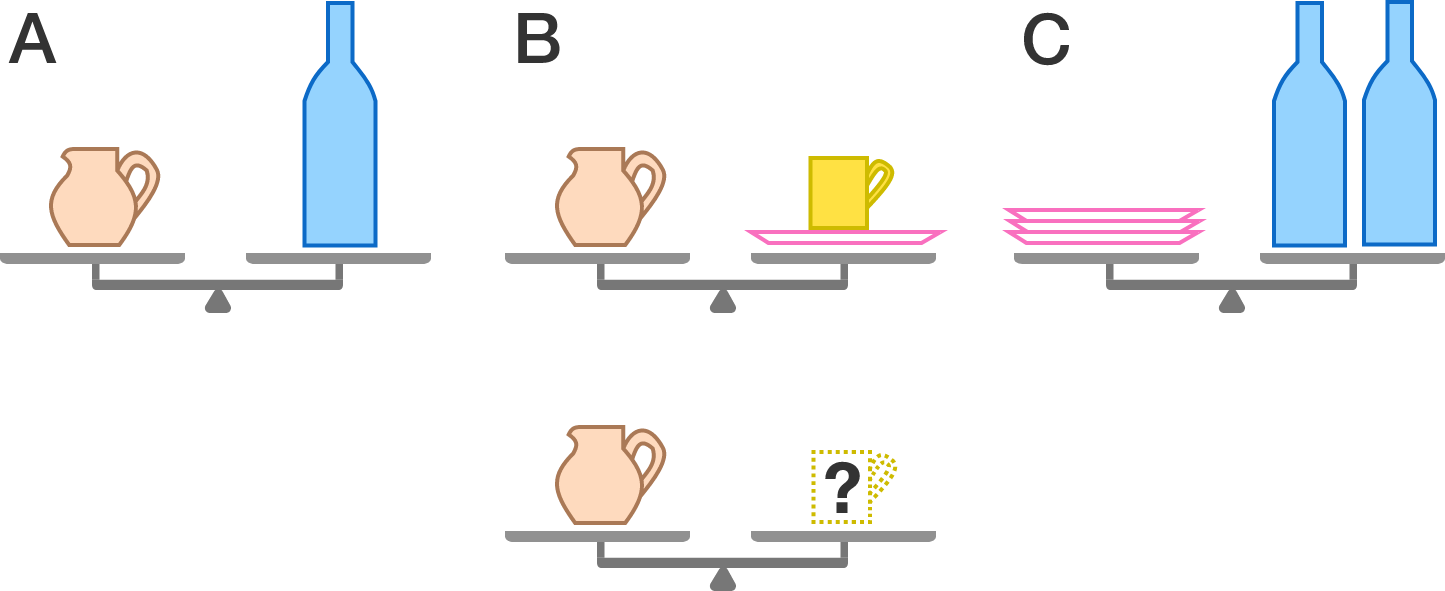In figure $A$, the jug on the left pan balances the bottle on the right pan.
In figure $B$, the jug alone balances a mug and a plate.
In figure $C$, three of these plates balance two bottles.

How many mugs will balance a jug?

Harry is going to be late to his class, so he needs to move as quickly as possible.

Here is a map of his institution, where the edges represent roads he could walk through, and the nodes of the same color are those between which he could teleport using the Floo Network: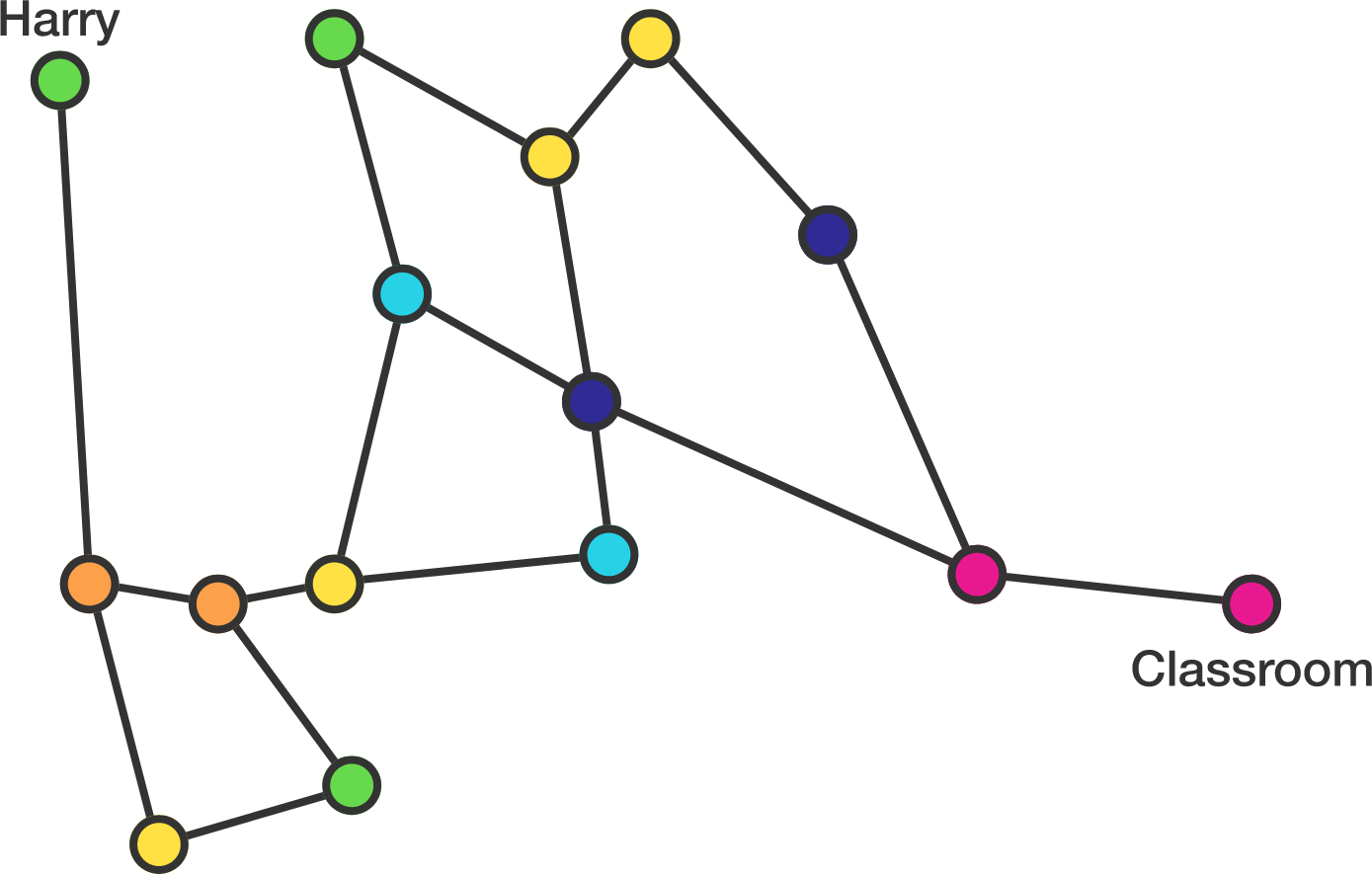What is the least number of roads he would need to walk through to get to the classroom?


Clarification: Teleportations happen instantaneously and thus are not included in the count.

Inspired by Christopher Boo

\begin{aligned} A &=& 1\times2\times\cdots \times 100 \\ B &=& 1\times2\times\cdots \times 200 \end{aligned}

It's obvious that $B\div A$ is an integer.
Is it also true that $B\div A \div A$ is still an integer?

×